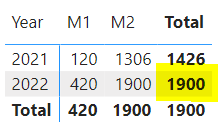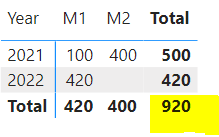cancel
Showing results for
Did you mean:Anonymous
Not applicable

## Incorrect Row Totals for Cumulative Sales

Hello!

I have a table where each row is already having cumulative sales in it and I have created a measure that displays cumulative sales of each model per part here as follows:

Cum_Sales =
Var Max_Date = MAX(Model_Sales[Date])
Var Latest_Sales = CALCULATE(SUM(Model_Sales[Cumulative Model Sales]), Model_Sales[Date] = Max_Date)
Return Latest_Sales

My cumulative sales for each model per part is all correct as is supposed to be. However, my totals of cumulative sales of all models per part is not coming accurate for row totals. When I try to see totals for any specific Part Number, then the total in row is either same as the cumulative sales of a speicifc Model number or is close to totals of all model numbers or is right total of all models. Is there anything that needs to be changed in the DAX shown above for cumulative sales while I am trying to see my cumulative sales of each model per part so that my Totals are correct?

I would like to see the Totals as total of cumulative sales of each Model for any selected Part. Totals of column is showing the right totals as that is the cumulative sales per part.  I have tried to create a mini sample to replicate the issue.

In below example shows incorrect totals of 1900 for 2022 & it should be 2320 instead.Its taking the value of only one model. Column totals are correct.In my real data, we have same issue as above. But there are also some rows with totals close to right total, but not exact right total. For example, if it is supposed be 1426 in above example for 2021, it shows 1418 or so. There are very few rows with right totals.

1 ACCEPTED SOLUTIONCommunity Support

Hi @Anonymous ,

Try this:

``````Cum_Sales_Tot V3 =
IF (
HASONEVALUE ( Dates[Year] ) || HASONEVALUE ( Part_to_Model[Model] ),
[Cum_Sales_Tot V2],
MAXX (
TOPN (
1,
SUMMARIZE ( Dates, Dates[Year], "Cum_Sales_V3", [Cum_Sales_Tot V2] ),
[Year]
),
[Cum_Sales_V3]
)
)
``````Best Regards,

Icey

If this post helps, then please consider Accept it as the solution to help the other members find it more quickly.

7 REPLIES 7Super User

@Anonymous

``````Cum_Sales_Tot V2 =
IF(ISINSCOPE(Part_to_Model[Model]),
[Cum_Sales],
SUMX(CROSSJOIN(DISTINCT(Dates[Year]), DISTINCT(Part_to_Model[Model])), [Cum_Sales]))``````Please accept the solution when done and consider giving a thumbs up if posts are helpful.  Contact me privately for support with any larger-scale BI needs, tutoring, etc.Anonymous
Not applicable

Thanks @AlB !

It works great for Row Total per Year as well as Column Total per Model except for the Total in the intersection of Row and Column. So, instead of 920 for our example, it should be 420Anonymous
Not applicableCommunity Support

Hi @Anonymous ,

Try this:

``````Cum_Sales_Tot V3 =
IF (
HASONEVALUE ( Dates[Year] ) || HASONEVALUE ( Part_to_Model[Model] ),
[Cum_Sales_Tot V2],
MAXX (
TOPN (
1,
SUMMARIZE ( Dates, Dates[Year], "Cum_Sales_V3", [Cum_Sales_Tot V2] ),
[Year]
),
[Cum_Sales_V3]
)
)
``````Best Regards,

Icey

If this post helps, then please consider Accept it as the solution to help the other members find it more quickly.Anonymous
Not applicable

Thanks @Icey! It works perfectly!

Thank you so much!

Thanks @AlB for making it simpler throughout and for solving it 95% for us.

Thank you both!Super User

Hi @Anonymous

Create a new measure based on [Sum_Sales] you already have:

``````Cum_Sales_Tot =
SUMX(CROSSJOIN(DISTINCT(Dates[Year]), DISTINCT(Part_to_Model[Model])), [Cum_Sales])``````Please accept the solution when done and consider giving a thumbs up if posts are helpful.  Contact me privately for support with any larger-scale BI needs, tutoring, etc.Anonymous
Not applicable

Thanks @AlB!

Row Totals are now correct and thats what I am looking for. However, the Column total was correct earlier showing only cumulative total per Model. Now, it shows column total as cumulative for all the years per model  instead of showing totals for only 2022 as that is cumulative value so far per model. How can we modify DAX and also, if this can be applicable even if months are selected as data is cumulative for each month.Announcements#### Exclusive opportunity for Women!

Join us for a free, hands-on Microsoft workshop led by women trainers for women where you will learn how to build a Dashboard in a Day!#### Power Platform Conference-Power BI and Fabric Sessions

Join us Oct 1 - 6 in Las Vegas for the Microsoft Power Platform Conference.Top Solution Authors
Top Kudoed Authors
Users online (4,114)